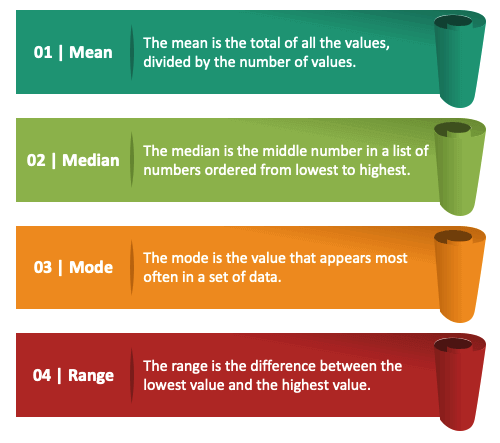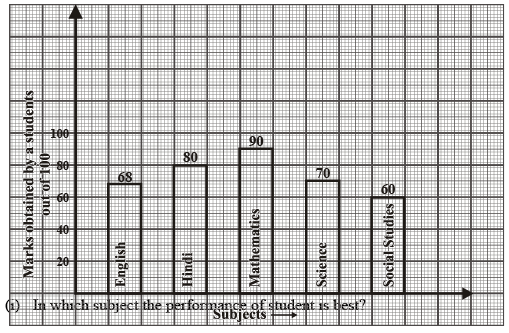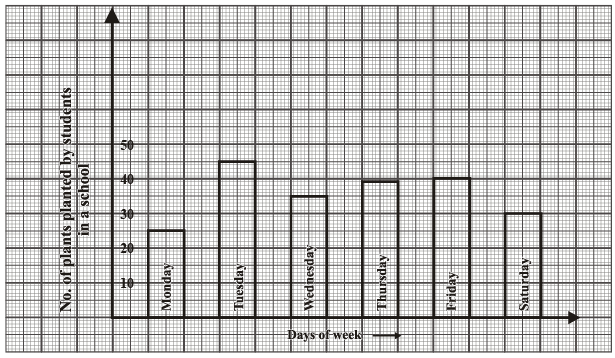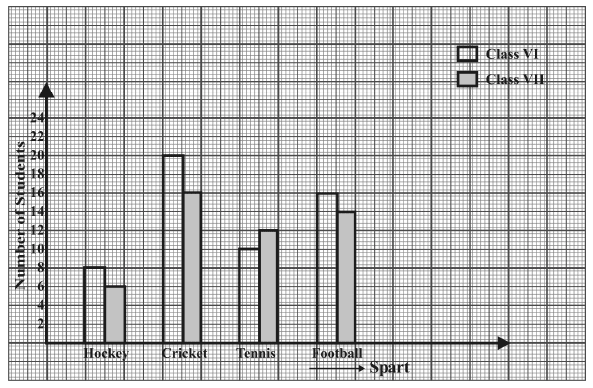NCERT Solutions: Data Handling- 3

# NCERT Solutions: Data Handling- 3 Notes | Study Mathematics (Maths) Class 7 - Class 7

## Document Description: NCERT Solutions: Data Handling- 3 for Class 7 2022 is part of Mathematics (Maths) Class 7 preparation. The notes and questions for NCERT Solutions: Data Handling- 3 have been prepared according to the Class 7 exam syllabus. Information about NCERT Solutions: Data Handling- 3 covers topics like and NCERT Solutions: Data Handling- 3 Example, for Class 7 2022 Exam. Find important definitions, questions, notes, meanings, examples, exercises and tests below for NCERT Solutions: Data Handling- 3.

Introduction of NCERT Solutions: Data Handling- 3 in English is available as part of our Mathematics (Maths) Class 7 for Class 7 & NCERT Solutions: Data Handling- 3 in Hindi for Mathematics (Maths) Class 7 course. Download more important topics related with notes, lectures and mock test series for Class 7 Exam by signing up for free. Class 7: NCERT Solutions: Data Handling- 3 Notes | Study Mathematics (Maths) Class 7 - Class 7
 1 Crore+ students have signed up on EduRev. Have you?

Ques 1: Fill in the blanks:
(i) Mean of first 5 natural numbers is ___________
(ii) Range fo the data 12, 15, 7, 9, 16, 18 is ___________
(iii) Median of the data 38, 40, 42, 48, 65, 72, 75, is ___________
(iv) Mode of the data 2, 3, 5, 6, 7, 3, 2, 9 is ___________
Ans: (i) 3
(ii) 11
(iii) 48
(iv) 3Mean, Median, Mode and Range

Ques 2: A die thrown once find the probability of the following:
(i) Getting a number greater than 5.
(ii) Getting a prime number.
(iii) Getting an even natural number.
(iv) Getting a number less than 4.
Ans: (i) 1/6
(ii) 1/2
(iii) 1/2
(iv) 1/2

Ques 3: If mean of 9, 5, 7, x, 6 is 6, find the value of x.
Ans: 3

Ques 4: What is the range of the these integers? 20, 6, 18, –15, –12, 0
Ans: 35

Ques 5: State whether the given statements are true or false
(i) Mean of the data is always from the given data.
(ii) The range of the data 2, 9, 6,5, 8 would change if 3 was added to each value in the data.
(iii) When a coin to tossed, there are two possible outcomes.
(iv) Probability of selecting you as a monitor within classs is greater than one.
Ans: (i) False
(ii) False
(iii) True
(iv) False

Ques 6: Study the bar graph given below and answer the questions that follow:(ii) Calcualte the average marks of the studnet.
(iii) If 75 and above marks denote a distiniction, then name the subjects in which the student got distinction.
(iv) Calcualte the percentage of marks the student got oug of 500.
Ans: (i) Mathematics
(ii) 73.6
(iii) Hindi, Mathematics
(iv) 73.6%

Ques 7: Observe the following data and answer the questions that follow:

 Days of the Week Mon Tue Wed Thu Fri Sat No. of plants planted by students in a shchool 25 45 35 38 40 30

(i) Draw a bar graph to represtn the above given in formation.
(ii) On which day of the week maximum number of plants planted by students.
(iii) Find the ratio of the minimum and maximum number of plants planted.
(iv) Find the total no. of plants planted.
Ans: (i)(ii) Tuesday
(iii) 5:9
(iv) 213

Ques 8: Study the double bar graphs given below and answer the following questions:(i) Which sport is liked the most by class VII students?
(ii) How many student are there in class VII if each student play only one sport?
(iii) How many students of class VI like hocky and football in all?
(iv) For which spart the number of students of class VII is more than that of class VI.
Ans: (i) Circket
(ii) 48
(iii) 24
(iv) Tennis

The document NCERT Solutions: Data Handling- 3 Notes | Study Mathematics (Maths) Class 7 - Class 7 is a part of the Class 7 Course Mathematics (Maths) Class 7.
All you need of Class 7 at this link: Class 7

## Mathematics (Maths) Class 7

168 videos|252 docs|45 tests
 Use Code STAYHOME200 and get INR 200 additional OFF

## Mathematics (Maths) Class 7

168 videos|252 docs|45 tests

Track your progress, build streaks, highlight & save important lessons and more!

,

,

,

,

,

,

,

,

,

,

,

,

,

,

,

,

,

,

,

,

,

;